Номер части: Журнал
ISSN: 2411-6467 (Print)
ISSN: 2413-9335 (Online)
Статьи, опубликованные в журнале, представляется читателям на условиях свободной лицензии CC BY-ND

# Comparison of Fuzzy Interference Systems for Documents Ranking Score

Науки и перечень статей вошедших в журнал:
DOI:
Дата публикации статьи в журнале:
Название журнала: Евразийский Союз Ученых — публикация научных статей в ежемесячном научном журнале, Выпуск: , Том: , Страницы в выпуске: -
Данные для цитирования: . Comparison of Fuzzy Interference Systems for Documents Ranking Score // Евразийский Союз Ученых — публикация научных статей в ежемесячном научном журнале. Технические науки. ; ():-.

1.Introduction

Fuzzy logic was first proposed in 1965 as a way to imprecise data by Loft Zadeh, professor at University of California.]. Fuzzy logic is methodology to represent and implement human’s knowledge about how to control a system . In fuzzy logic, knowledge can be captured in terms of rules and linguistic variables.

Fuzzy inference systems (FIS) are rule-based systems with concepts and operations associated with fuzzy set theory and fuzzy logic . These systems are mappings from an input space to an output space. Therefore,they allow constructing structures that can be used to generate responses outputs) to certain stimulations (inputs), based on stored knowledge on how the responses and stimulations related. The knowledge is stored in the form of a rule base, i.e. a set of rules that express the relationsbetween inputs of the system and expected outputs. Sometimes this knowledge is obtained by eliciting information from specialists, in which case these systems are usually known as fuzzy expert systems .

Fuzzy logic, which reproduce the approximate reasoning process of the human mind byrepresenting  knowledge via linguistic if-then rules, allow for precise output inference starting from imprecise input . The aim of this paper is to describe different types of fuzzy logic systems that could be used for the evaluation the document ranking score.

Usually an information retrieval system returns large result sets and the users must spend considerable time to find items that are really relevant. Moreover, documents are retrieved when they contain the index terms specified in the queries . However, this approach will neglect other relevant documents that do not contain the index terms specified in the user’s queries. Number of document retrieval from Automatic Information Retrieval. Multi agent modeling (as will be explained in section 2.3)  areused in this paper for ranking score to make decision .This decision making helps user to choose the best documents with his quires

In this paper, we use fuzzy logic to evaluate number of documents (ranking score). The evaluation is using cosinesimilarity methodbetween the query as a search engine and the retrieval document result from Automatic Information Retrieval Multi agent modeling.

The rest of the paper is organized as follows. Section 2 explains the methodology. Section3 illustrates proposed work.Section 4 explainsexperimental evaluation example. Sections 5 discuss the results.Section 6 conclude the paper.

1. Methodology

2.1 Fuzzy Inference System

Fuzzy inference is the process of formulating the mapping from given input(s) to output(s) using fuzzy logic. A fuzzy inference system as shown in figure (1) .It has four main parts: (1) Fuzzification interface simply modifies and converts inputs into suitable linguistic values so that can be compared to the rules in the rule base. (2) Knowledge base containing Rule base, holds the knowledge in the form of a set of rule andalso a number of a database which defines the membership functions of the fuzzy sets used in fuzzy rules. (3) Inference mechanism, evaluates which rules are relevant at current time and then decides what the output should be. (4)Defuzzification interface, converts the conclusions reached by the inference mechanism into crisp output , .

Fuzzy if-then rules or fuzzy condition allstatements are expressions of the form: If x is A Then y is B.where, x and y are input and output linguistic variables. A and B are labels of the fuzzy sets characterized by appropriate membership functions. A is the premise and B is the consequent parts of the fuzzy rule. Fuzzy values A and B are described by the membership functions. The forms of membership functions are different and problem depended.

The steps of fuzzy inference operations upon fuzzy IF–THEN rules performed by FISs are described as follows

1. Compare the input variables with the membership functions on the antecedent part to obtain the membership values of each linguistic label (this step is often called fuzzification).
2. Combine (usually prod or min) the membership values on the premise part to get firing strength (weight) of each rule.
3. Generate the qualified consequents (either fuzzy or crisp) of each rule depending on the firing strength.
4. Aggregate the qualified consequents to produce a crisp output (This step is called defuzzification).

The most common types of fuzzy inference that have been introduced in the literature and applied to different applications are Mamdani and Sugeno type models , ,  and   The most fundamental difference between Mamdani-type FIS and Sugeno-type FIS is described in the following section.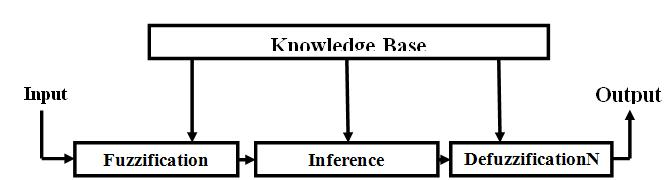Figure 1 Fuzzy Inference System 

2.2MAMDANI-TYPE FIS VS. SUGENO-TYPE FIS

The most fundamental difference between Mamdani type FIS and Sugeno type FIS is the way the crisp output is generated from the fuzzy inputs. While Mamdani FIS uses the technique of defuzzification of a fuzzy output, Sugeno FIS uses weighted average to compute the crisp output. Therefore in Sugeno FIS the defuzzification process is bypassed . Other difference is that Mamdani FIS has output membership functions whereas Sugeno FIS has no output membership functions. Mamdani FIS is less flexible in system design in comparison to Sugeno FIS. Mamdani method is widely accepted for capturing expert knowledge. It allows us to describe the expertise in more intuitive, more human-like manner. However, Mamdani-type FIS entails a substantial computational burden. On the other hand, Sugeno method is computationally efficient and works well with optimization and adaptive techniques, which makes it very attractive in control problems, particularly for dynamic nonlinear systems. These adaptive techniques can be used to customize the membership functions so that fuzzy system best models the data , .

2.3 Multi agent system modeling

The purpose of the multi-agent system is to aid users in searching and retrieving information available on the World Wide Web. A system devoted to perform automatic information retrieval might encompass four  main steps: (i) Searching the world wide web with keyword, (ii) Extracting the required information from web sources (iii) Mining the texts that extracted from the web by using automatically rapid miner (iv)  And   at the end Storing output result of multi-document using term weight in database (excel file). Agents are java agent development environment (JADE).JADE agents capable of (i) interacting exchanging FIPA-ACL messages, (ii) sharing a common ontology in accordance with the actual application, and (iii) exhibiting a specific behavior according to their role

2.4Vector Space Model

TF-IDF is the most common weighting method used to describe documents in the Vector Space Model (VSM). It is composed of Term frequency and Inverse Document Frequency. Term frequency is a local weight of a term on a document achieved by counting the number of times a particular term occurs in a text document. The higher frequency of a term on a document is the more relevant term in that particular document.  Normalized term frequency of term t in document d shown in Equation (1) as the ratio of frequency of each term in the document to the maximum term frequency in that document. Therefore, the greater the frequency of particular terms in the document, the greater the normalized frequency of such term in the document.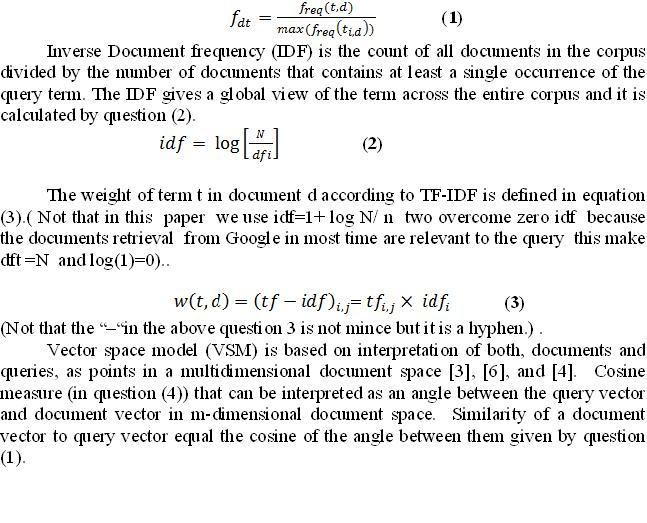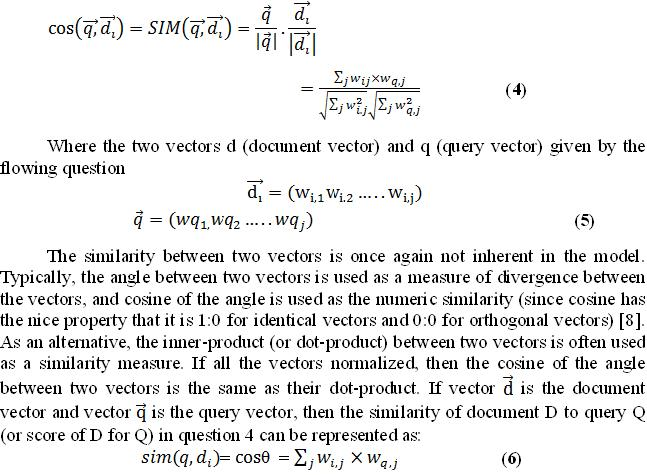2.5 Fuzzy Rules base

In a FIS, a rule base is constructed to control the output variable. A fuzzy rule is a simple IF-THEN rule with a condition and a conclusion.  In the following rules will be derived from a common knowledge about information retrieval and the tf-idf of w (d,t) and  w(q,t))  weighting .

If a query  t  term in a document w(d ,t) has high tf and idf measures , so term weight (TW)  or called weight of term W( as in equation 3)   is the product of them (tf*idf) , the document is likely to be highly score  as shown in question (3,6 )  will be high .

The rule are the following:

1. If (w (q, t1) is high) and (w (t1, d)is high) then (cosine (q,d) is score high)
2. If (w (q, t1) is low) and (w (t1, d) is low) then (cosine (q,d) is score low)
3. If (w (t2, d) is high) and (w (q, t2) is high) then (cosine (q,d) is score high)
4. If (w (t2, d) is low) and (w (q, t2) is low) then (cosine (q,d) is score low)

Where W (t ,q)  is weight of term  (tf-idf)  of the term in the query (Wq,j) and W(t, d) is the weight of term  (tf-idf)  of the term j  in the documenti (Wi, j) As in questions (6 ), ( 3).

1. Proposed work:

We will sum upon this part as shown in block diagram in figure (2)whatwill bedone:

• At first documents are retrieved by using Automatic Information Retrieval Multi agent modeling all the documents which retrieved is stored in matrix with terms after tokenizing, stemming levels and illuminate the stop–word.
• Extract weight of term (tf-idf) of the terms according to the query (key word enter by the user in multi–agent model). This term represents by using question 3 and called w(t,d) or wij as  in question  Also it can be compute the weight of term w (q,t)  as  the same in equation  3 (tf-idf) or wqj in question  4 of the query terms (keywords). (Not that all weight of terms must be normalized).
• The weight of terms of the document and quires as described in step 3 enter as input to the fuzzy inference system.
• The fuzzy inference system (Mamdani and Sugeno type) operate as illustrated in steps in section (2.1) to calculate the similarity between document and query to evaluate the document ranking score to make decision of the high and low score.
• At the last compare all the result which will obtain from Mamdani and Sugeno FIS type to kwon which of them is best develop the document ranking score to take decision.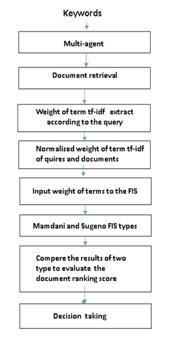Figure 2 Block diagram of proposed work

1. Experimental evaluation example:

The experiment evaluation was carried out in MATLAB platform, Mamdani-type FIS and Sugeno- type FIS and a sample of the documents retrieval from multi-agent. The experiment was run to evaluate the ranking score of relevant documents using question (6) and keywords query as (computer science.)

4.1 Evaluation by using Mamdani-type FIS

The proposed FIS for the evaluation documents  rankingscore consists of four inputs  ( two  for weigh term of document and two for weight term in the query) as shown in figure 3.1 w(t1,d),w(q,t1),w(t2,d),w(q,t2). The system has one output that indicates score of document. Each of the selected input and output variables is described by a set of two linguistic fuzzy values (low and high) defined by triangle membership function, thus allowing the fuzzification procedure to convert the measured numerical value into one of the fuzzy values.Figure3.2 shows one of the input w(q,t2)  triangle membership function .and figure 4shows output  score (cosine similarity) triangle membership functions. In the experiment the input processed by Mamdani-type fuzzy inference system using triangle membership function and rules as described in section (2.5) and as was shown in figure .3.1.The input for the defuzzification process was the aggregate output fuzzy set (of sum after applied rules in section 2.5) and the output set was a single number(centroid value) as shown in figure 4A and figure 4 b.The document in figure 4 f was highest score (centroid value 0.666) but the document in figure 4awas the lowest score (centroid value 0.573) .  The plots obtained after simulating Mamdani-type of FIS for document similarity cosine score were shown in figures. 4 c and figure 4 d .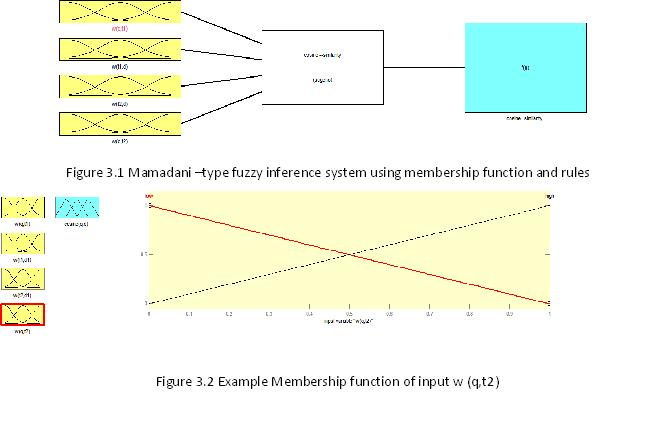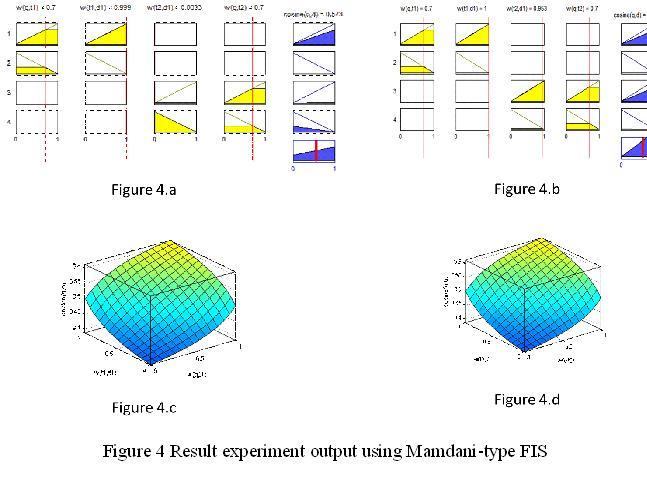4.2 Evaluation by using Sugeno -type FIS

The initial steps and the setting of Sugeno-type FIS aresame as of Mamdni-type FIS. It also consists of four  inputs( two  for weigh term of document and two for weight term in the query) as shown in figure 5  w(t1,d),w(q,t1),w(t2,d),w(q,t2). and produces one output thatindicates the  similarity or the ranking score ( cosine) . Each of the selected inputvariables is described by a set of two linguistic fuzzy values,defined by triangle membershipfunctions in the case of Mamdani-type fuzzy inference system(as already shown inFigs3.2). Unlike the output value range of the Mamdani-typefuzzy inference system, the range of Sugeno-type output isbetween 0 and 1.The output of this system can only be eitherconstant or linear in this FIS, so two linguistic fuzzy values for the output are “Low”, and “High” which can be constant low score 0 and high score 1. The rule base for Sugenotype FIS is the same as for Mamdani-type FIS as shown in section 2.5.

In the experiment the input processed by Sugeno–type fuzzy inference system using triangle membership function and rules as described in section (2.5) and as was shown in figure (3.1). The output set was a single number(weighted average) as shown in figure 6and figure 6b  .The document in figure 6 f was highest score (weighted average0.999) , but the document in figure 6awas the lowest score (centroid value 0.714) .The plots obtained after simulating Mamdani-type of FIS for document similarity cosine score were shown in figures6.c and figure  6 d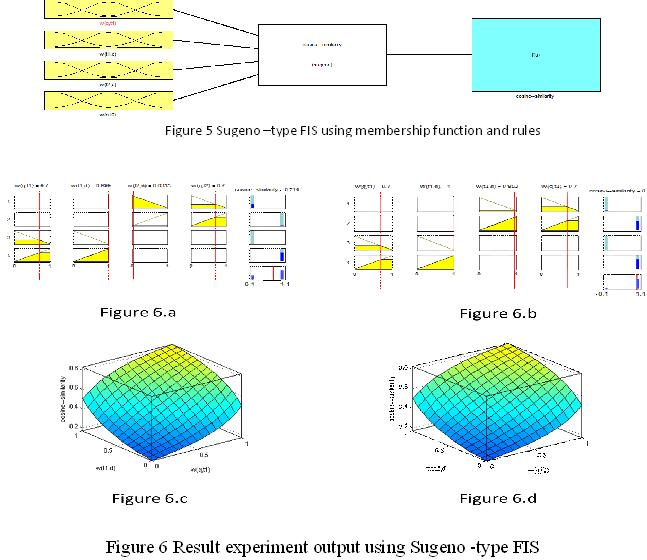5.RESULTS DISCUSSIONS

As illustrates in the figures above, both inference systems (Mamdani-type and Sugeno-type) responded to operational changes of the inputs and obtained output same result of document ranking score. However, the Sugeno-typeFIS has betterresults (cosine score) than that of the Mamdani-type FIS for documents evaluation.  From these simulation results. The differences between Mamdani type FIS and Sugeno type FIS are: (1). the way the crisp output is generated from the fuzzy inputs. (2). Mamdani FIS has output membership functions whereas Sugeno FIS has no output membership functions.

1. CONCLUSION

It has been concluded from this paper that for the evaluate document ranking score using similarity method (cosine), Mamdani-type FIS and Sugeno-type FIS works similarly. Membership functions and rules are same for both the FIS, only difference is that output membership functions for Sugeno-type FIS can only be either constant or linear and also the crisp output is generated in different ways for both the FIS.Sugeno-type FIS is better results better than Mamdani-type. Both the models are simulated using 4rulesand four input membership Functions. Also only one output value (centroid value) is used.in the case of Mamdani-type FIS and (average weight value) in the case of Sugeno-type FIs for document ranking score.

References

Addis.A Study and Development of Novel Techniques for Hierarchical Text       Categorization: Dissertation,University of Cagliari, 2010.

 Kamboj.V and Kaur. A Comparison of Constant SUGENO-Type and MAMDANI-Type Fuzzy Inference System for Load Sensor:International Journal of Soft Computing and Engineering (IJSCE) ISSN: 2231-2307, 3: (2), May 2013.

 Kaur. A and Kaur.A Comparison of Mamdani Fuzzy Model and Neuro Fuzzy Model for Air Conditioning System : International Journal of Computer Science and Information Technologies, 3 :(2),2012,  3593-3596.

 Manning. C.,Raghava. P and Schütze.H Introduction to Information Retrieval: Cambridge University Press, 2008 .

Ross.T Fuzzy Logic with Engineering Applications:John Wiley and Sons, 2010.

 Salton.G , Wong. A and Yang.C. S A Vector Space Model for Automatic Indexing: Communications of the ACM , 18:(11),1975,  pp. 613-620.

Shleeg. A and  Ellabib. I  Comparison of Mamdani and Sugeno Fuzzy Interference Systems for the Breast Cancer Risk : World Academy of Science, Engineering and Technology, International Journal of Computer, Information, Systems and Control Engineering  , 7:(10), 2013.

Yen. J and Langari.R Fuzzy Logic intelligence, control & information: Pearson Education, India, 2004.[schema type=»book» name=»Comparison of Fuzzy Interference Systems for Documents Ranking Score» description=» Document ranking score is the method of evaluate documents retrieved from web using multi-agent model. In this paper, Fuzzy Inference Systems are used for the evaluation documents using Mamdani-type and Sugeno-type models. This paper at first presents a multi-agent system aimed at creating a generic multi-agent architecture aimed at retrieving, filtering, and reorganizing information according to the users’ interests in a web-based environment by using a powerful agent framework, java agent development environment (JADE). Second, the paper outlines the basic difference between Mamdani-type Fuzzy Inference Systems and Sugeno- type Fuzzy Inference Systems. Both Mamdani-type fuzzy inference system and Sugeno-type fuzzy inference system are simulated using MATLAB fuzzy logic toolbox.» author=»Ark Andreev, Galina Ivanova, Marwa Shouman» publisher=»БАСАРАНОВИЧ ЕКАТЕРИНА» pubdate=»2017-05-26″ edition=»ЕВРАЗИЙСКИЙ СОЮЗ УЧЕНЫХ_ 30.01.2015_01(10)» ebook=»yes» ]

Список литературы:

##### euroasia
Записи созданы 9819

## ИСПОЛЬЗОВАНИЕ ЖЕЛЕЗОСОДЕРЖАЩЕЙ ПИЩЕВОЙ ДОБАВКИ В ПРОИЗВОДСТВЕ ХЛЕБА

Начните вводить, то что вы ищите выше и нажмите кнопку Enter для поиска. Нажмите кнопку ESC для отмены.

Вернуться наверх